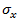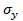Goseeko blog# What is Correlation? Why it is so important?

## Overview

Correlation is the measurement of the strength of a linear relationship between two variables say x and y.

In other words, we define it as if the change in one variable affects a change in other variables, then these two variables are said to be correlated.

For example:

1.     The correlation between a person’s income and expenditures.

2.     As the temperature goes up, the demand for ice cream also goes up.

## Types of correlation

Positive correlation- When both variables move in the same direction, or if the increase in one variable results in a corresponding increase in the other one is called positive correlation.

Negative correlation- When one variable increases and other decreases or vise-versa, then the variables said to be negatively correlated.

No correlation- When two variables are independent and do not affect each other then there will be no correlation between the two and said to be uncorrelated.

Note- (Perfect correlation)- When a variable changes constantly with the other variable, then these two variables are said to be perfectly correlated.

## Scatter plots or dot diagrams

A scatter or dot diagram is used to check the correlation between the two variables.

It is the simplest method to represent bivariate data.

When the dots in the diagram are very close to each other, then we can say that there is a fairly good correlation.

If the dots are scattered then we get a poor correlation.

## Karl Pearson’s coefficient of correlation

Karl Person’s coefficient of correlation is also called product-moment correlation coefficient.

It is denoted by ‘r’, and defined as-

Hereandare the standard deviations of these series.

Alternate formula-

Note-

• Correlation coefficient always lies between -1 and +1.
• Correlation coefficient is independent of change of origin and scale.
• If the two variables are independent then correlation coefficient between them is zero.

## Solved example

Example: The data given below is about the marks obtained by a student and hours she studied.

Find the correlation coefficient between hours and marks obtained.

Solution:

Let hours = x and marks = y

Karl Person’s coefficient of correlation is given by-

The correlation coefficient between hours and marks obtained is-

r = 0.98